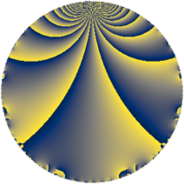Properties

 Label 100.2.aLevel $100$ Weight $2$ Character orbit 100.a Rep. character $\chi_{100}(1,\cdot)$ Character field $\Q$ Dimension $1$ Newform subspaces $1$ Sturm bound $30$ Trace bound $0$

Related objects

Defining parameters

 Level: $$N$$ $$=$$ $$100 = 2^{2} \cdot 5^{2}$$ Weight: $$k$$ $$=$$ $$2$$ Character orbit: $$[\chi]$$ $$=$$ 100.a (trivial) Character field: $$\Q$$ Newform subspaces: $$1$$ Sturm bound: $$30$$ Trace bound: $$0$$

Dimensions

The following table gives the dimensions of various subspaces of $$M_{2}(\Gamma_0(100))$$.

Total New Old
Modular forms 24 1 23
Cusp forms 7 1 6
Eisenstein series 17 0 17

The following table gives the dimensions of the cuspidal new subspaces with specified eigenvalues for the Atkin-Lehner operators and the Fricke involution.

$$2$$$$5$$FrickeDim.
$$-$$$$+$$$$-$$$$1$$
Plus space$$+$$$$0$$
Minus space$$-$$$$1$$

Trace form

 $$q + 2 q^{3} - 2 q^{7} + q^{9} + O(q^{10})$$ $$q + 2 q^{3} - 2 q^{7} + q^{9} - 2 q^{13} + 6 q^{17} - 4 q^{19} - 4 q^{21} - 6 q^{23} - 4 q^{27} + 6 q^{29} - 4 q^{31} - 2 q^{37} - 4 q^{39} + 6 q^{41} + 10 q^{43} + 6 q^{47} - 3 q^{49} + 12 q^{51} + 6 q^{53} - 8 q^{57} + 12 q^{59} + 2 q^{61} - 2 q^{63} - 2 q^{67} - 12 q^{69} - 12 q^{71} - 2 q^{73} + 8 q^{79} - 11 q^{81} - 6 q^{83} + 12 q^{87} - 6 q^{89} + 4 q^{91} - 8 q^{93} - 2 q^{97} + O(q^{100})$$

Decomposition of $$S_{2}^{\mathrm{new}}(\Gamma_0(100))$$ into newform subspaces

Label Dim. $$A$$ Field CM Traces A-L signs $q$-expansion
$a_{2}$ $a_{3}$ $a_{5}$ $a_{7}$ 2 5
100.2.a.a $1$ $0.799$ $$\Q$$ None $$0$$ $$2$$ $$0$$ $$-2$$ $-$ $+$ $$q+2q^{3}-2q^{7}+q^{9}-2q^{13}+6q^{17}+\cdots$$

Decomposition of $$S_{2}^{\mathrm{old}}(\Gamma_0(100))$$ into lower level spaces

$$S_{2}^{\mathrm{old}}(\Gamma_0(100)) \cong$$ $$S_{2}^{\mathrm{new}}(\Gamma_0(20))$$$$^{\oplus 2}$$$$\oplus$$$$S_{2}^{\mathrm{new}}(\Gamma_0(50))$$$$^{\oplus 2}$$